# SSAT Middle Level Math : How to find the square root

## Example Questions

1 3 Next →

### Example Question #21 : How To Find The Square Root

If a square garden has an area of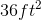, what is the length of its sides?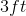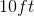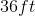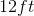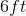Explanation:

To find the area of a square, you must square the length of its sides.  So to move backwards to find the side length, you must find the square root of the area.  The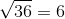so the side length is.

### Example Question #22 : How To Find The Square Root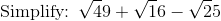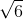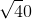Explanation:

We must first simplify all of the square roots.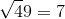Because 7 times 7 =49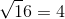Because 4 times 4 =16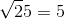Because 5 times 5 = 25

Now we plug all of these into the original equation to get: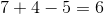### Example Question #23 : How To Find The Square Root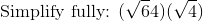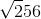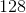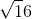Explanation:

First we must simplify the square roots.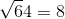Because 8 times 8 = 64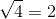Because 2 times 2 = 4

Now we have: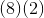When two parentheses are beside each other, that means muliply.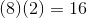### Example Question #2121 : Ssat Middle Level Quantitative (Math)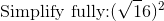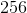Explanation:

First we must simplify what is inside the brackets.Because 4 times 4 = 16.

Now we have: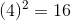Again, because 4 times 4 =16.

We can also see that the square root and the squared are inverse operations which mean that they undo each other to get the same thing that we started with.

### Example Question #23 : How To Find The Square Root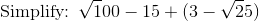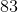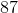Explanation:We must first simplify what is in ().

Since the square root of 25 = 5, we can replace that with 5 to get.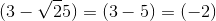So everything in the () is replaced with (-2)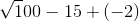We know that the square root of 100 equals 10, so we can replace that.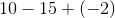Which simplifies to become: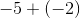Which givs us the final answer which is:### Example Question #24 : How To Find The Square Root

What is the square root of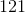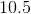Explanation:

A square root is a product of a number multiplied by itself. You need to figure out which number multiplied by itself is equal to. You can use trial and error if you don't know it immediately.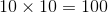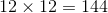If you test these two numbers, you can determine the answer is a number between 10 and 12, because 121 is between 100 and 144.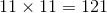### Example Question #25 : How To Find The Square Root

Which of the following numbers has a square root between 18 and 19?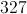All of these numbers have square roots between 18 and 19.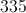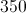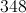All of these numbers have square roots between 18 and 19.

Explanation:

For the square root of a number to fall between 18 and 19, the number has to fall between the squares of these numbers - that is:

If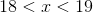, then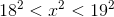, or, equivalently,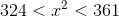All four choices fall in this range.

1 3 Next →

### All SSAT Middle Level Math Resources